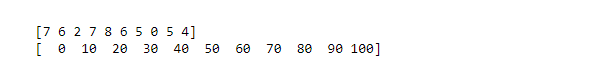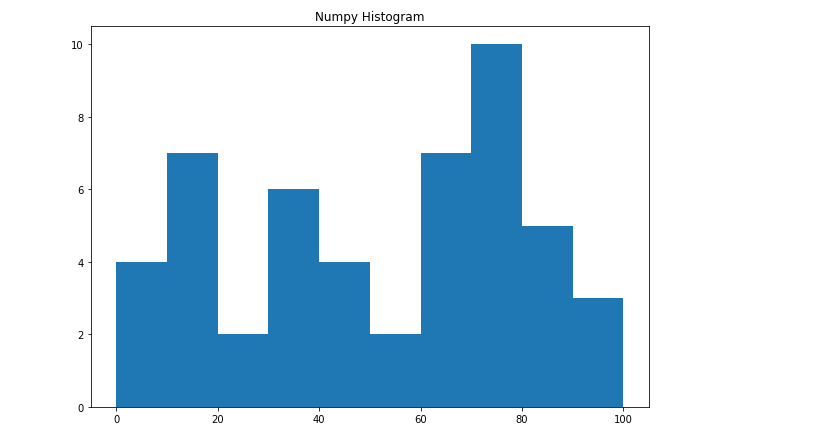# NumPy.histogram() Method in Python

A histogram is the best way to visualize the frequency distribution of a dataset by splitting it into small equal-sized intervals called bins. The Numpy histogram function is similar to the `hist()` function of matplotlib library, the only difference is that the Numpy histogram gives the numerical representation of the dataset while the `hist()` gives graphical representation of the dataset.

## Creating Numpy Histogram

Numpy has a built-in `numpy.histogram()` function which represents the frequency of data distribution in the graphical form. The rectangles having equal horizontal size corresponds to class interval called bin and variable height corresponding to the frequency.

Syntax:

numpy.histogram(data, bins=10, range=None, normed=None, weights=None, density=None)

Attributes of the above function are listed below:

Attribute Parameter
data array or aequence of array to be plotted
bins int or sequence of str defines number of equal width bins in a range, default is 10
range optional parameter sets lower and upper range of bins
normed optional parameter same as density attribute, gives incorrect result for unequal bin width
weights optional parameter defines array of weights having same dimensions as data
density optional parameter if False result contain number of sample in each bin, if True result contain probability density function at bin

The function has two return values hist which gives the array of values of the histogram, and edge_bin which is an array of float datatype containing the bin edges having length one more than the hist.

Example:

 `# Import libraries ` `import` `numpy as np  ` ` `  ` `  `# Creating dataset ` `a ``=` `np.random.randint(``100``, size ``=``(``50``)) ` ` `  `# Creating histogram ` `np.histogram(a, bins ``=` `[``0``, ``10``, ``20``, ``30``, ``40``, ` `                        ``50``, ``60``, ``70``, ``80``, ``90``, ` `                        ``100``]) ` ` `  `hist, bins ``=` `np.histogram(a, bins ``=` `[``0``, ``10``,  ` `                                     ``20``, ``30``, ` `                                     ``40``, ``50``, ` `                                     ``60``, ``70``, ` `                                     ``80``, ``90``, ` `                                     ``100``])  ` ` `  `# printing histogram ` `print``() ` `print` `(hist)  ` `print` `(bins)  ` `print``() `

Output:## Graphical representation

The above numeric representation of histogram can be converted into a graphical form.The `plt()` function present in `pyplot` submodule of Matplotlib takes the array of dataset and array of bin as parameter and creates a histogram of the corresponding data values.

Example:

 `# import libraries ` `from` `matplotlib ``import` `pyplot as plt  ` `import` `numpy as np   ` ` `  ` `  `# Creating dataset ` `a ``=` `np.random.randint(``100``, size ``=``(``50``)) ` ` `  `# Creating plot ` `fig ``=` `plt.figure(figsize ``=``(``10``, ``7``)) ` ` `  `plt.hist(a, bins ``=` `[``0``, ``10``, ``20``, ``30``, ` `                    ``40``, ``50``, ``60``, ``70``, ` `                    ``80``, ``90``, ``100``])  ` ` `  `plt.title(``"Numpy Histogram"``)  ` ` `  `# show plot ` `plt.show() `

Output:My Personal Notes arrow_drop_upCheck out this Author's contributed articles.

If you like GeeksforGeeks and would like to contribute, you can also write an article using contribute.geeksforgeeks.org or mail your article to contribute@geeksforgeeks.org. See your article appearing on the GeeksforGeeks main page and help other Geeks.

Please Improve this article if you find anything incorrect by clicking on the "Improve Article" button below.

Article Tags :

1

Please write to us at contribute@geeksforgeeks.org to report any issue with the above content.